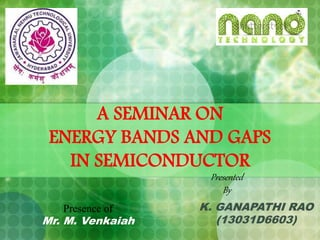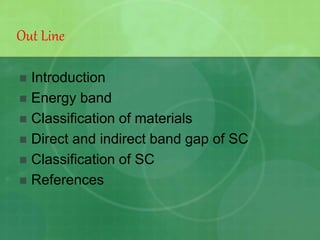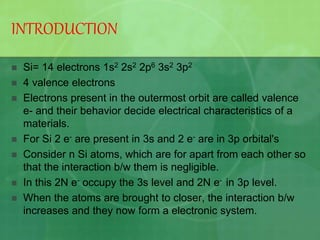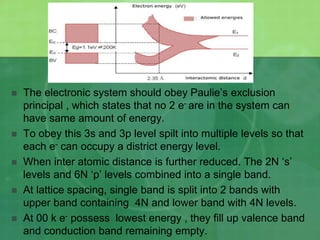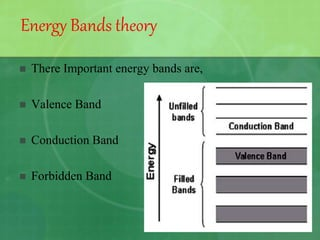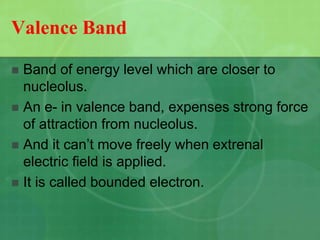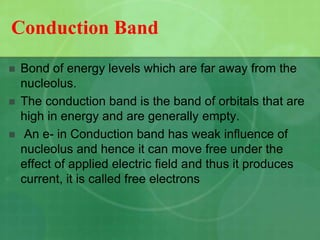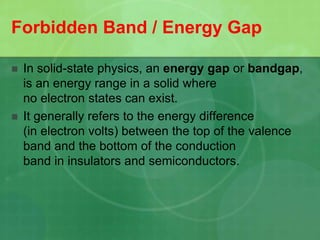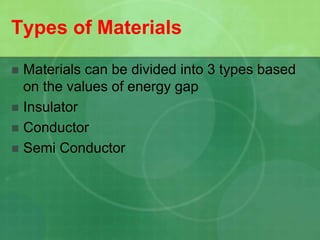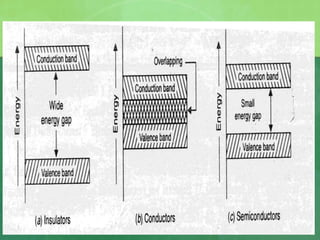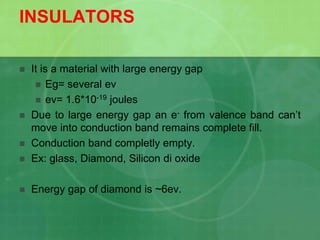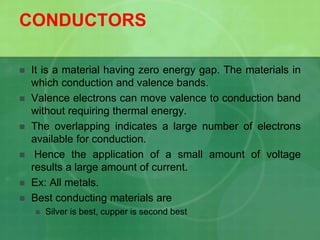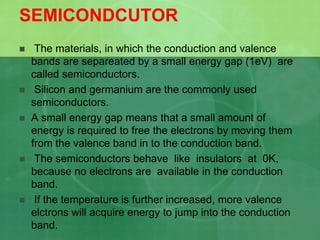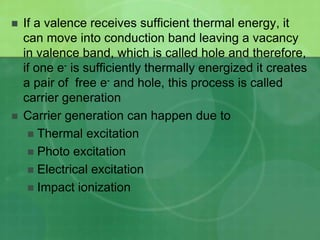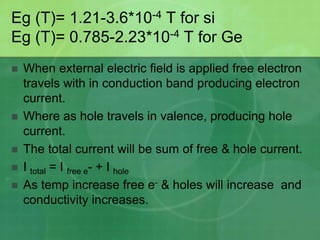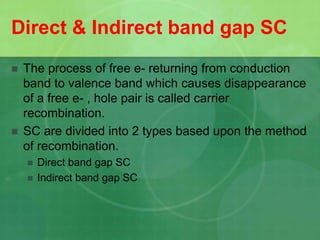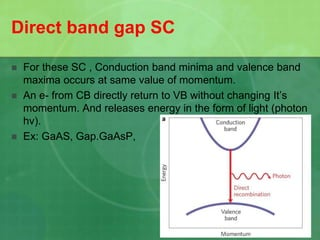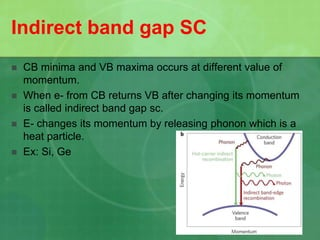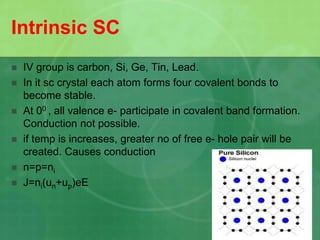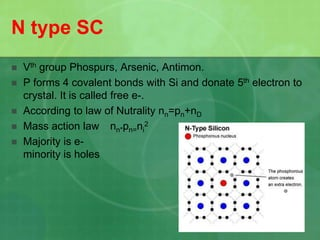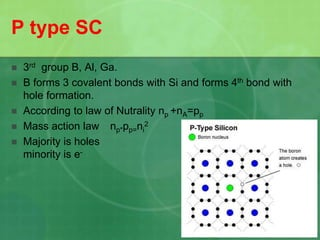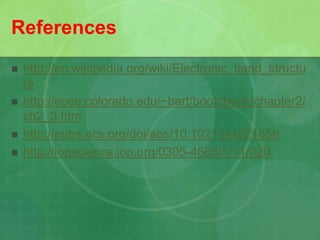1 de 23

### Energy bands and gaps in semiconductor

1. A SEMINAR ON ENERGY BANDS AND GAPS IN SEMICONDUCTOR K. GANAPATHI RAO (13031D6603) Presented By Presence of Mr. M. Venkaiah
2.  Introduction  Energy band  Classification of materials  Direct and indirect band gap of SC  Classification of SC  References Out Line
3. INTRODUCTION  Si= 14 electrons 1s2 2s2 2p6 3s2 3p2  4 valence electrons  Electrons present in the outermost orbit are called valence e- and their behavior decide electrical characteristics of a materials.  For Si 2 e- are present in 3s and 2 e- are in 3p orbital's  Consider n Si atoms, which are for apart from each other so that the interaction b/w them is negligible.  In this 2N e- occupy the 3s level and 2N e- in 3p level.  When the atoms are brought to closer, the interaction b/w increases and they now form a electronic system.
4.  The electronic system should obey Paulie’s exclusion principal , which states that no 2 e- are in the system can have same amount of energy.  To obey this 3s and 3p level spilt into multiple levels so that each e- can occupy a district energy level.  When inter atomic distance is further reduced. The 2N ‘s’ levels and 6N ‘p’ levels combined into a single band.  At lattice spacing, single band is split into 2 bands with upper band containing 4N and lower band with 4N levels.  At 00 k e- possess lowest energy , they fill up valence band and conduction band remaining empty.
5.  There Important energy bands are,  Valence Band  Conduction Band  Forbidden Band Energy Bands theory
6. Valence Band  Band of energy level which are closer to nucleolus.  An e- in valence band, expenses strong force of attraction from nucleolus.  And it can’t move freely when extrenal electric field is applied.  It is called bounded electron.
7. Conduction Band  Bond of energy levels which are far away from the nucleolus.  The conduction band is the band of orbitals that are high in energy and are generally empty.  An e- in Conduction band has weak influence of nucleolus and hence it can move free under the effect of applied electric field and thus it produces current, it is called free electrons
8. Forbidden Band / Energy Gap  In solid-state physics, an energy gap or bandgap, is an energy range in a solid where no electron states can exist.  It generally refers to the energy difference (in electron volts) between the top of the valence band and the bottom of the conduction band in insulators and semiconductors.
9. Types of Materials  Materials can be divided into 3 types based on the values of energy gap  Insulator  Conductor  Semi Conductor
10. INSULATORS  It is a material with large energy gap  Eg= several ev  ev= 1.6*10-19 joules  Due to large energy gap an e- from valence band can’t move into conduction band remains complete fill.  Conduction band completly empty.  Ex: glass, Diamond, Silicon di oxide  Energy gap of diamond is ~6ev.
11. CONDUCTORS  It is a material having zero energy gap. The materials in which conduction and valence bands.  Valence electrons can move valence to conduction band without requiring thermal energy.  The overlapping indicates a large number of electrons available for conduction.  Hence the application of a small amount of voltage results a large amount of current.  Ex: All metals.  Best conducting materials are  Silver is best, cupper is second best
12. SEMICONDCUTOR  The materials, in which the conduction and valence bands are separeated by a small energy gap (1eV) are called semiconductors.  Silicon and germanium are the commonly used semiconductors.  A small energy gap means that a small amount of energy is required to free the electrons by moving them from the valence band in to the conduction band.  The semiconductors behave like insulators at 0K, because no electrons are available in the conduction band.  If the temperature is further increased, more valence elctrons will acquire energy to jump into the conduction band.
13.  If a valence receives sufficient thermal energy, it can move into conduction band leaving a vacancy in valence band, which is called hole and therefore, if one e- is sufficiently thermally energized it creates a pair of free e- and hole, this process is called carrier generation  Carrier generation can happen due to  Thermal excitation  Photo excitation  Electrical excitation  Impact ionization
14. Eg (T)= 1.21-3.6*10-4 T for si Eg (T)= 0.785-2.23*10-4 T for Ge  When external electric field is applied free electron travels with in conduction band producing electron current.  Where as hole travels in valence, producing hole current.  The total current will be sum of free & hole current.  I total = I free e- + I hole  As temp increase free e- & holes will increase and conductivity increases.
15. Direct & Indirect band gap SC  The process of free e- returning from conduction band to valence band which causes disappearance of a free e- , hole pair is called carrier recombination.  SC are divided into 2 types based upon the method of recombination.  Direct band gap SC  Indirect band gap SC
16. Direct band gap SC  For these SC , Conduction band minima and valence band maxima occurs at same value of momentum.  An e- from CB directly return to VB without changing It’s momentum. And releases energy in the form of light (photon hv).  Ex: GaAS, Gap.GaAsP,
17. Indirect band gap SC  CB minima and VB maxima occurs at different value of momentum.  When e- from CB returns VB after changing its momentum is called indirect band gap sc.  E- changes its momentum by releasing phonon which is a heat particle.  Ex: Si, Ge
18. Intrinsic SC  IV group is carbon, Si, Ge, Tin, Lead.  In it sc crystal each atom forms four covalent bonds to become stable.  At 00 , all valence e- participate in covalent band formation. Conduction not possible.  if temp is increases, greater no of free e- hole pair will be created. Causes conduction  n=p=ni  J=ni(un+up)eE
19. N type SC  Vth group Phospurs, Arsenic, Antimon.  P forms 4 covalent bonds with Si and donate 5th electron to crystal. It is called free e-.  According to law of Nutrality nn=pn+nD  Mass action law nn*pn=ni 2  Majority is e- minority is holes
20. P type SC  3rd group B, Al, Ga.  B forms 3 covalent bonds with Si and forms 4th bond with hole formation.  According to law of Nutrality np +nA=pp  Mass action law np*pp=ni 2  Majority is holes minority is e-
21. References  http://en.wikipedia.org/wiki/Electronic_band_structu re  http://ecee.colorado.edu/~bart/book/book/chapter2/ ch2_3.htm  http://pubs.acs.org/doi/abs/10.1021/jz402165b  http://iopscience.iop.org/0305-4608/5/11/020